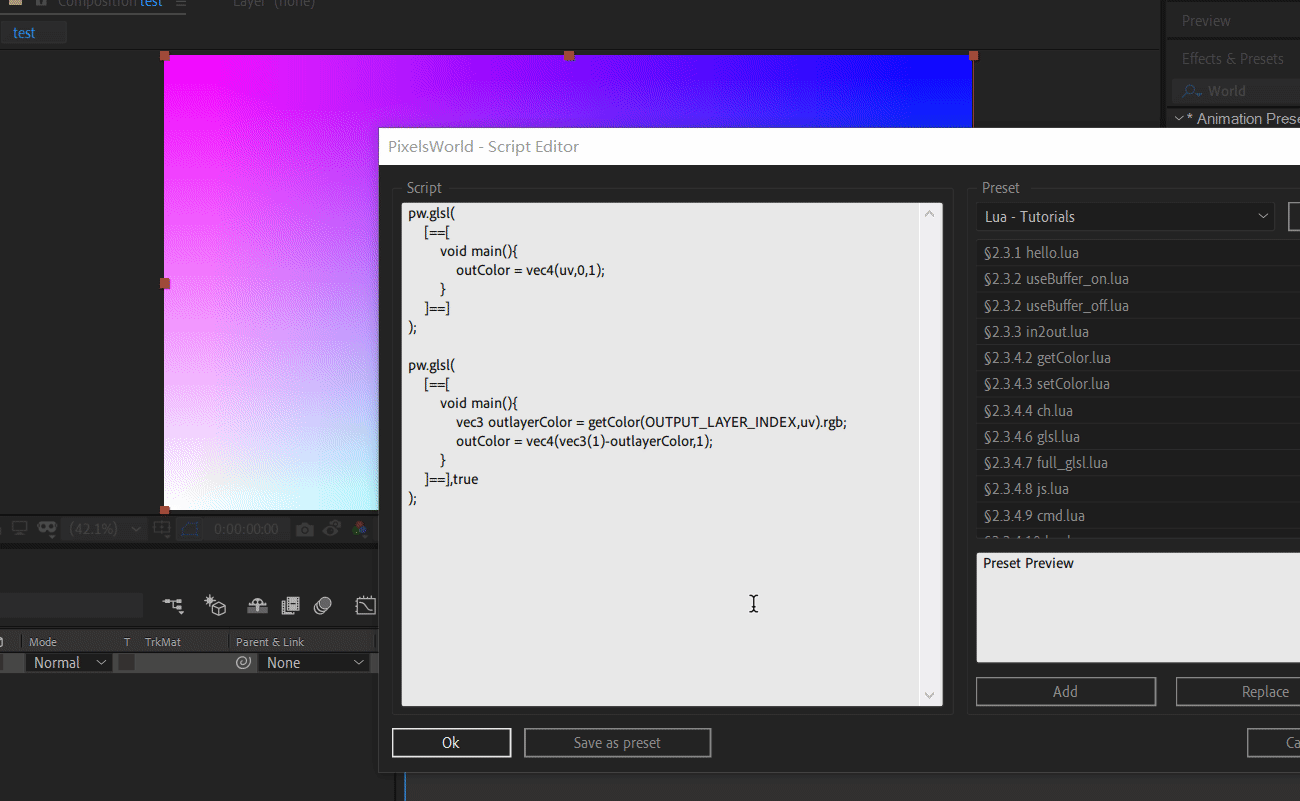# 在lua中运行GLSL

## 用法

### pw.glsl(GLSLcode[, useBuffer])

• GLSLcode: 字符串，源分段代码。 如果您输入的代码可以在 GLSL渲染模式下运行， 则可在此处直接使用 (此处不支持来自shadertoy.com的代码)
• useBuffer : 布尔值，可选参数。默认为false。 如果为true，PixelsWorld会生成临时内存来储存渲染结果。GLSL渲染完毕后，临时储存器中的数据会覆盖输出层的像素数据。

useBuffer 设置为true的情况：
• 从输出层重新读取像素 (即, GLSL代码中含有 `getColor(OUTPUT_LAYER_INDEX,uv)` )
• 希望渲染速度减慢

如果每帧仅渲染一次GLSL，则此项可以忽略

1. 您可以使用`[==[``]==]`符号包裹住多行代码。

useBuffer_on.lua

``````pw.glsl(
[==[
void main(){
outColor = vec4(uv,0,1);
}
]==]
);

pw.glsl(
[==[
void main(){
vec3 outlayerColor = getColor(OUTPUT_LAYER_INDEX,uv).rgb;
outColor = vec4(vec3(1)-outlayerColor,1);
}
]==],true
);
``````

useBuffer_off.lua

``````pw.glsl(
[==[
void main(){
outColor = vec4(uv,0,1);
}
]==]
);

pw.glsl(
[==[
void main(){
vec3 outlayerColor = getColor(OUTPUT_LAYER_INDEX,uv).rgb;
outColor = vec4(vec3(1)-outlayerColor,1);
}
]==],false
);
``````